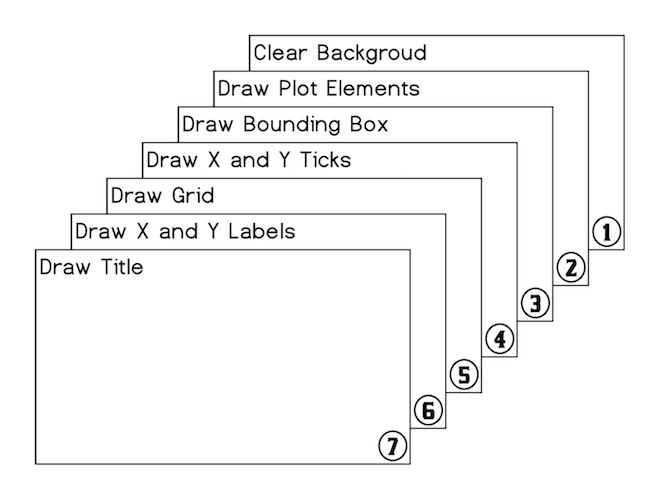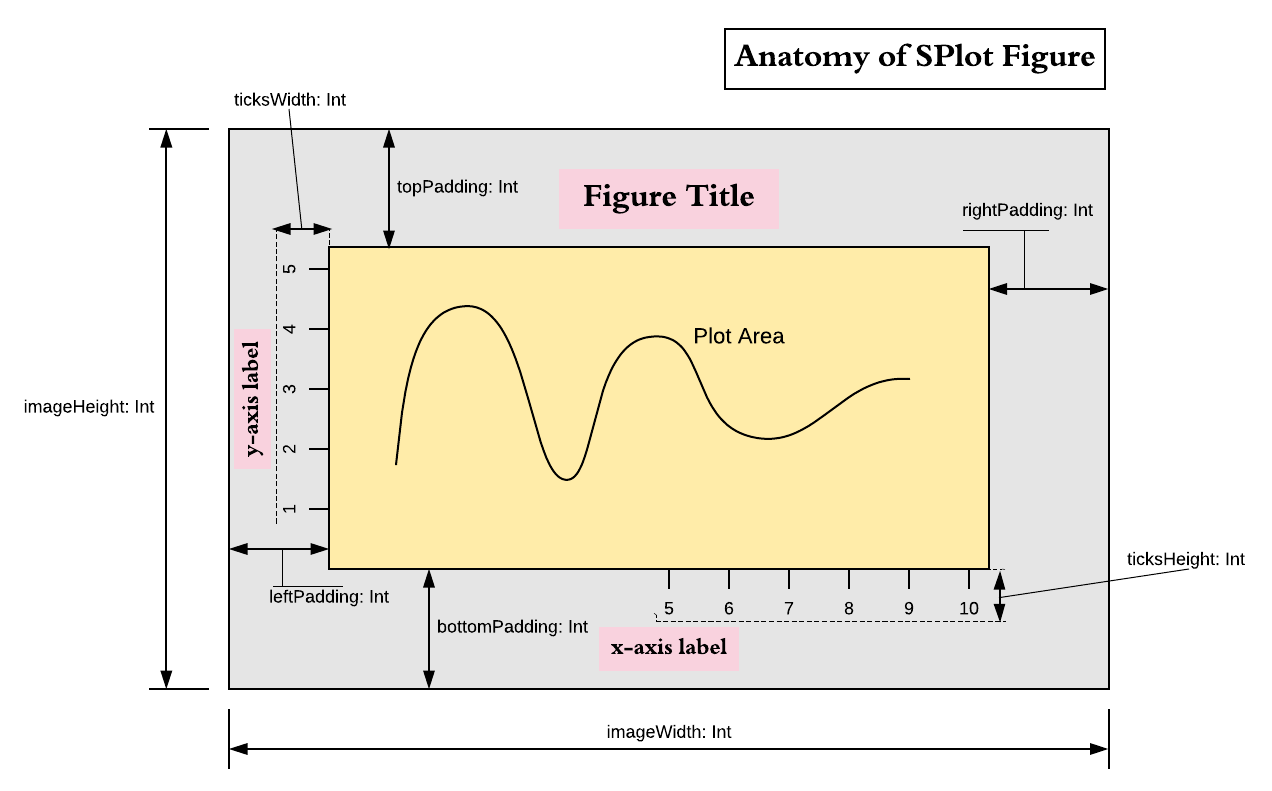# Architecture

## Overview

Central element of the SPlot library is object `Figure`. Plot elements, such as lines and labels and so on, are added to it and then Figure can either display an interactive window or it can be used to generate image object, which can then be saved on disk. By itself `Figure` does almost nothing. It is really a skeleton which stitches together different objects required to form an image or a window. All these objects, that are part of Figure, are responsible for drawing themselves. To do so they receive appropriate context and parameters. Some of the elements can appear multiple times in Figure, such as line plots and labels. They are added by using `Figure::add(...)` method. Other elements, referenced by Figure, exist in singular form. These objects refer to things like function that draws bounding box (a.k.a. spines of the figure) or function that draws ticks, etc. They are supplied to the `Figure` constructor with certain default values that can be changed to draw plots very differently. For example, you could change the way bounding box is plotted to draw it with rounded corners or not draw bounding box at all. Note that default objects/functions passed to `Figure` constructor already allow certain customization, but creating your own custom variants gives complete freedom to modify figure drawing behaviour.

## Pipeline Outline

After all plot elements are added to the `Figure` users can either call `crateImage(width, height)` function to create an image or call `show(width, height)` to display an interactive window. In both cases blank `BufferedImage` is created and drawing pipeline is applied to it. Steps of the pipeline are shown in the figure below.All of these steps, 1 through 7, are performed by externally supplied functions. Plot elements drawn in stage 2 are the only ones that users usually explicitly add to the figure. All other steps are performed by singularly referenced functions for which default instances are already supplied to `Figure` during construction.

## Figure Constructor Parameters

Besides objects and functions responsible for fulfilling steps of the drawing pipeline users can pass following parameters to the `Figure` constructor:

``````name:          String = "Figure"
title:         String = ""
titleFont:     Font = Font.decode("Times-20")
xLabel:        String = ""
yLabel:        String = ""
bgColor:       Color = Color.WHITE
antialiasing:  Boolean = true
showGrid:      Boolean = false
domain:        Option[(Double, Double)] = None
range:         Option[(Double, Double)] = None
xTicks:        (Double, Double) => Seq[(Double, String)] = Ticks.ticks10
yTicks:        (Double, Double) => Seq[(Double, String)] = Ticks.ticks5
``````

Based on these parameters, prior to executing drawing pipeline, `DrawingContext` object is created. It contains important figure dimensions such as `imageWidth, imageHeight, leftPadding, rightPadding, topPadding, bottomPadding`, and a set of functions and other objects required for pipeline steps to draw themselves. Following image provides visual illustration of figure properties encapsulated in `DrawingContext`:## Pipeline Step Functions

Pipeline steps are performed by the following functions:

1. Clear Background.
``````def backgroudPlotter(ctx: DrawingContext, bgColor: Color): Unit
``````
2. Draw plot elements. Plot elements have three forms: trait `Plot` for drawing lines and shapes, `Label` for drawing text labels, and `CompositePlotElement` that contains sequence of the other two element types.
``````trait PlotElement

trait Plot extends PlotElement {
def draw(ctx: DrawingContext): Unit
}

trait Label extends PlotElement {
def draw(g2: Graphics2D, atPosition: (Int, Int)): Unit
}

case class CompositePlotElement(plotElements: Seq[Either[Plot, Label]])
extends PlotElement
``````

Plot elements are drawn in the order they were added to the figure and therefore can obscure each other.

3. Draw bounding box
``````def borderPlotter(ctx: DrawingContext, color: Color): Unit
``````
4. Draw ticks, first on x-axis and then on y-axis, using following two functions:
``````def xTicksPlotter(ctx: DrawingContext, ticks: Seq[(Double, String)]): Int
``````

and

``````def yTicksPlotter(ctx: DrawingContext, ticks: Seq[(Double, String)]): Int
``````

These functions are responsible for drawing the ticks and their labels. They must return height/length of the ticks together with labels. Functions `xLabelPlotter` and `yLabelPlotter` use these values to find proper placement for `x` and `y` labels.

5. Draw grid
``````def gridPlotter(ctx: DrawingContext, xTicks: Seq[Int], yTicks: Seq[Int]): Unit
``````
6. Draw `x` and `y` labels using following two functions.
``````def xLabelPlotter(ctx: DrawingContex, ticksLength: Int, text: String): Unit

def yLabelPlotter(ctx: DrawingContex, ticksLength: Int, text: String): Unit
``````
7. Draw figure title
``````def titlePlotter(DrawingContext, String, Font): Unit
``````